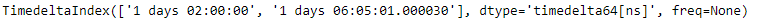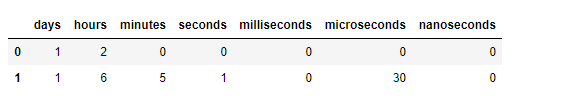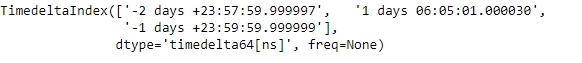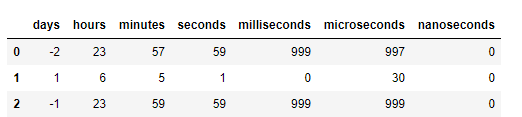Related Articles
Python | Pandas TimedeltaIndex.components
• Last Updated : 29 Dec, 2018

Python is a great language for doing data analysis, primarily because of the fantastic ecosystem of data-centric python packages. Pandas is one of those packages and makes importing and analyzing data much easier.

Pandas` TimedeltaIndex.components` attribute return a dataframe of the components (days, hours, minutes, seconds, milliseconds, microseconds, nanoseconds) of the Timedeltas.

Syntax: TimedeltaIndex.components

Return : a DataFrame

Example #1: Use `TimedeltaIndex.components` attribute to return a dataframe of the components of the Timedeltas.

 `# importing pandas as pd``import` `pandas as pd`` ` `# Create the TimedeltaIndex object``tidx ``=` `pd.TimedeltaIndex(data ``=``[``'1 days 02:00:00'``, ``                                ``'1 days 06:05:01.000030'``])`` ` `# Print the TimedeltaIndex``print``(tidx)`

Output :Now we want to return a dataframe of the components of the tidx object.

 `# return a dataframe constructed from``# the components of the Timedelta``tidx.components`

Output :As we can see in the output, the `TimedeltaIndex.components` attribute has returned a dataframe constructed from the components of the Timedelta

Example #2: Use `TimedeltaIndex.components` attribute to return a dataframe of the components of the Timedeltas.

 `# importing pandas as pd``import` `pandas as pd`` ` `# Create the TimedeltaIndex object``tidx ``=` `pd.TimedeltaIndex(data ``=``[``'-1 days 2 min 3us'``, ``'1 days 06:05:01.000030'``,``                                                 ``'-1 days + 23:59:59.999999'``])`` ` `# Print the TimedeltaIndex``print``(tidx)`

Output :Now we want to return a dataframe of the components of the tidx object.

 `# return a dataframe constructed from``# the components of the Timedelta``tidx.components`

Output :As we can see in the output, the `TimedeltaIndex.components` attribute has returned a dataframe constructed from the components of the Timedelta

Attention geek! Strengthen your foundations with the Python Programming Foundation Course and learn the basics.

To begin with, your interview preparations Enhance your Data Structures concepts with the Python DS Course.

My Personal Notes arrow_drop_up